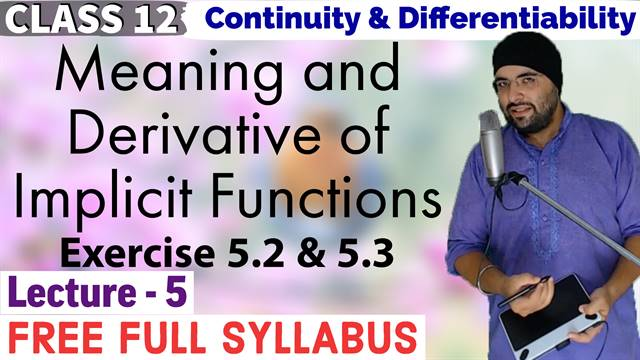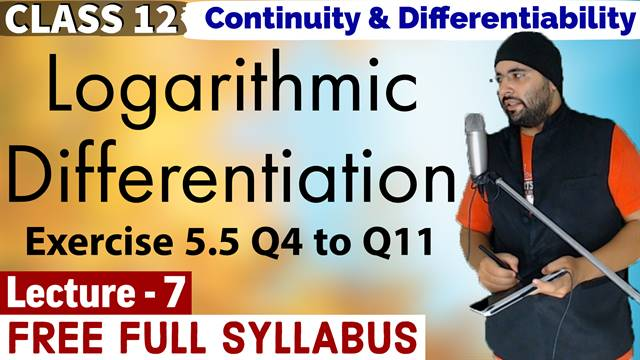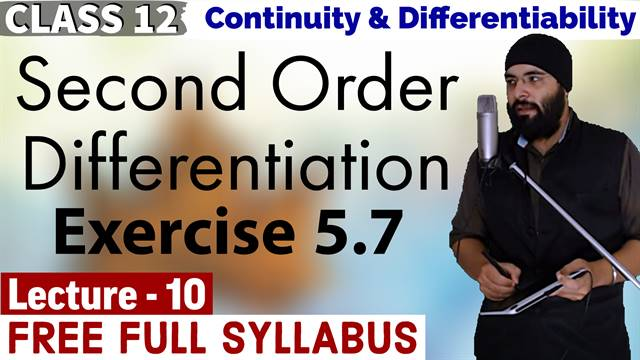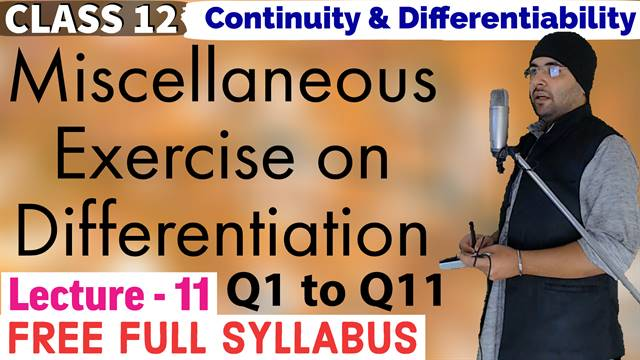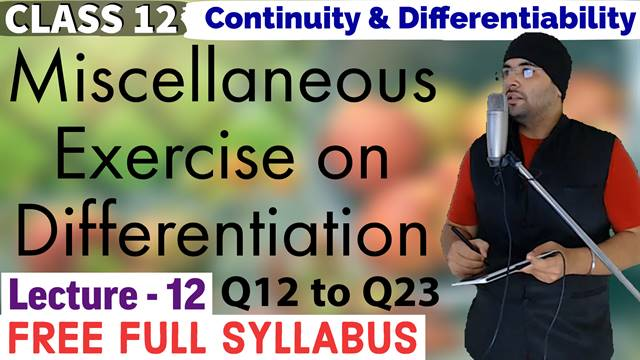# 5. Continuity And Differentiability

A real valued function is continuous at a point in its domain if the limit of the function at that point equals the value of the function at that point. A function is continuous if it is continuous on the whole of its domain.

Sum, difference, product and quotient of continuous functions are continuous. i.e., if f and g are continuous functions, then
(f ± g) (x) = f(x) ± g(x) is continuous.
(f . g) (x) = f(x) . g (x) is continuous.
\left( \frac{f}{g} \right)(x) = \frac{f(x)}{g(x)} ( \text{ wherever }  g(x) \ne 0 ) is continuous.

Every differentiable function is continuous, but the converse is not true.

Chain rule is rule to differentiate composites of functions.

Logarithmic differentiation is a powerful technique to differentiate functions of the form f(x) = [u (x)]^{v (x)} . Here both f(x) and u(x) need to be positive for this technique to make sense.

## Select Lecture